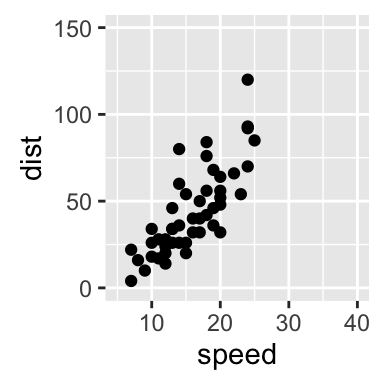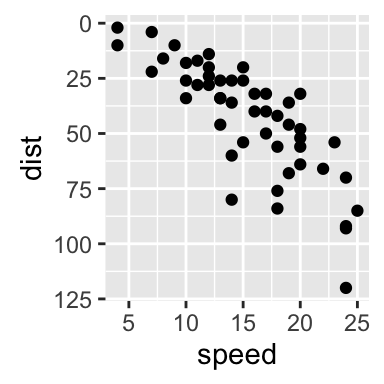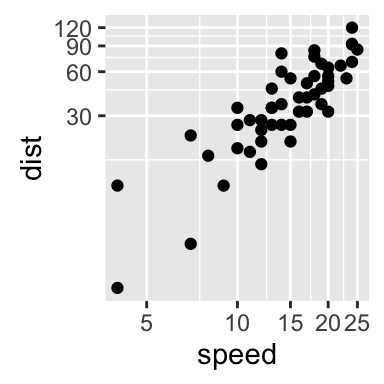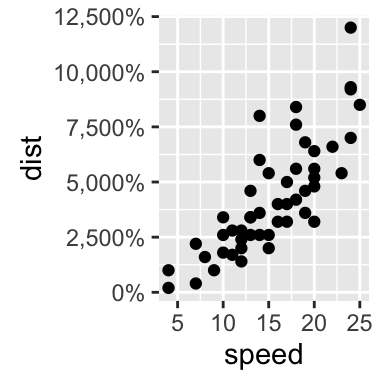# ggplot2 axis scales and transformations

This R tutorial describes how to modify x and y axis limits (minimum and maximum values) using ggplot2 package. Axis transformations (log scale, sqrt, …) and date axis are also covered in this article.

# Prepare the data

ToothGrowth data is used in the following examples :

``````# Convert dose column dose from a numeric to a factor variable
ToothGrowth\$dose <- as.factor(ToothGrowth\$dose)
``````##    len supp dose
## 1  4.2   VC  0.5
## 2 11.5   VC  0.5
## 3  7.3   VC  0.5
## 4  5.8   VC  0.5
## 5  6.4   VC  0.5
## 6 10.0   VC  0.5``````

Make sure that dose column is converted as a factor using the above R script.

# Example of plots

``````library(ggplot2)
# Box plot
bp <- ggplot(ToothGrowth, aes(x=dose, y=len)) + geom_boxplot()
bp
# scatter plot
sp<-ggplot(cars, aes(x = speed, y = dist)) + geom_point()
sp``````# Change x and y axis limits

There are different functions to set axis limits :

• xlim() and ylim()
• expand_limits()
• scale_x_continuous() and scale_y_continuous()

## Use xlim() and ylim() functions

To change the range of a continuous axis, the functions xlim() and ylim() can be used as follow :

``````# x axis limits
sp + xlim(min, max)
# y axis limits
sp + ylim(min, max)``````

min and max are the minimum and the maximum values of each axis.

``````# Box plot : change y axis range
bp + ylim(0,50)
# scatter plots : change x and y limits
sp + xlim(5, 40)+ylim(0, 150)``````## Use expand_limts() function

Note that, the function expand_limits() can be used to :

• quickly set the intercept of x and y axes at (0,0)
• change the limits of x and y axes
``````# set the intercept of x and y axis at (0,0)
sp + expand_limits(x=0, y=0)
# change the axis limits
sp + expand_limits(x=c(0,30), y=c(0, 150))``````## Use scale_xx() functions

It is also possible to use the functions scale_x_continuous() and scale_y_continuous() to change x and y axis limits, respectively.

The simplified formats of the functions are :

``````scale_x_continuous(name, breaks, labels, limits, trans)
scale_y_continuous(name, breaks, labels, limits, trans)``````

• name : x or y axis labels
• breaks : to control the breaks in the guide (axis ticks, grid lines, …). Among the possible values, there are :
• NULL : hide all breaks
• waiver() : the default break computation
• a character or numeric vector specifying the breaks to display
• labels : labels of axis tick marks. Allowed values are :
• NULL for no labels
• waiver() for the default labels
• character vector to be used for break labels
• limits : a numeric vector specifying x or y axis limits (min, max)
• trans for axis transformations. Possible values are “log2”, “log10”, …

The functions scale_x_continuous() and scale_y_continuous() can be used as follow :

``````# Change x and y axis labels, and limits
sp + scale_x_continuous(name="Speed of cars", limits=c(0, 30)) +
scale_y_continuous(name="Stopping distance", limits=c(0, 150))``````# Axis transformations

## Log and sqrt transformations

Built in functions for axis transformations are :

• scale_x_log10(), scale_y_log10() : for log10 transformation
• scale_x_sqrt(), scale_y_sqrt() : for sqrt transformation
• scale_x_reverse(), scale_y_reverse() : to reverse coordinates
• coord_trans(x =“log10”, y=“log10”) : possible values for x and y are “log2”, “log10”, “sqrt”, …
• scale_x_continuous(trans=‘log2’), scale_y_continuous(trans=‘log2’) : another allowed value for the argument trans is ‘log10’

These functions can be used as follow :

``````# Default scatter plot
sp <- ggplot(cars, aes(x = speed, y = dist)) + geom_point()
sp
# Log transformation using scale_xx()
# possible values for trans : 'log2', 'log10','sqrt'
sp + scale_x_continuous(trans='log2') +
scale_y_continuous(trans='log2')
# Sqrt transformation
sp + scale_y_sqrt()
# Reverse coordinates
sp + scale_y_reverse() ``````The function coord_trans() can be used also for the axis transformation

``````# Possible values for x and y : "log2", "log10", "sqrt", ...
sp + coord_trans(x="log2", y="log2")``````## Format axis tick mark labels

Axis tick marks can be set to show exponents. The scales package is required to access break formatting functions.

``````# Log2 scaling of the y axis (with visually-equal spacing)
library(scales)
sp + scale_y_continuous(trans = log2_trans())
# show exponents
sp + scale_y_continuous(trans = log2_trans(),
breaks = trans_breaks("log2", function(x) 2^x),
labels = trans_format("log2", math_format(2^.x)))``````Note that many transformation functions are available using the scales package : log10_trans(), sqrt_trans(), etc. Use help(trans_new) for a full list.

Format axis tick mark labels :

``````library(scales)
# Percent
sp + scale_y_continuous(labels = percent)
# dollar
sp + scale_y_continuous(labels = dollar)
# scientific
sp + scale_y_continuous(labels = scientific)``````## Display log tick marks

It is possible to add log tick marks using the function annotation_logticks().

Note that, these tick marks make sense only for base 10

The Animals data sets, from the package MASS, are used :

``````library(MASS)
``````##                     body brain
## Mountain beaver     1.35   8.1
## Cow               465.00 423.0
## Grey wolf          36.33 119.5
## Goat               27.66 115.0
## Guinea pig          1.04   5.5
## Dipliodocus     11700.00  50.0``````

The function annotation_logticks() can be used as follow :

``````library(MASS) # to access Animals data sets
library(scales) # to access break formatting functions
# x and y axis are transformed and formatted
p2 <- ggplot(Animals, aes(x = body, y = brain)) + geom_point() +
scale_x_log10(breaks = trans_breaks("log10", function(x) 10^x),
labels = trans_format("log10", math_format(10^.x))) +
scale_y_log10(breaks = trans_breaks("log10", function(x) 10^x),
labels = trans_format("log10", math_format(10^.x))) +
theme_bw()
# log-log plot without log tick marks
p2
# Show log tick marks
p2 + annotation_logticks()  ``````Note that, default log ticks are on bottom and left.

To specify the sides of the log ticks :

``````# Log ticks on left and right
p2 + annotation_logticks(sides="lr")
# All sides
p2+annotation_logticks(sides="trbl")``````

Allowed values for the argument sides are :

• t : for top
• r : for right
• b : for bottom
• l : for left
• the combination of t, r, b and l

# Format date axes

The functions scale_x_date() and scale_y_date() are used.

## Create some time serie data

``````df <- data.frame(
date = seq(Sys.Date(), len=100, by="1 day")[sample(100, 50)],
price = runif(50)
)
df <- df[order(df\$date), ]
``````##          date      price
## 33 2016-09-21 0.07245190
## 3  2016-09-23 0.51772443
## 23 2016-09-25 0.05758921
## 43 2016-09-26 0.99389551
## 45 2016-09-27 0.94858770
## 29 2016-09-28 0.82420890``````

## Plot with dates

``````# Plot with date
dp <- ggplot(data=df, aes(x=date, y=price)) + geom_line()
dp``````## Format axis tick mark labels

Load the package scales to access break formatting functions.

``````library(scales)
# Format : month/day
dp + scale_x_date(labels = date_format("%m/%d")) +
theme(axis.text.x = element_text(angle=45))
# Format : Week
dp + scale_x_date(labels = date_format("%W"))
# Months only
dp + scale_x_date(breaks = date_breaks("months"),
labels = date_format("%b"))``````Note that, since ggplot2 v2.0.0, date and datetime scales now have date_breaks, date_minor_breaks and date_labels arguments so that you never need to use the long scales::date_breaks() or scales::date_format().

## Date axis limits

US economic time series data sets (from ggplot2 package) are used :

``head(economics)``
``````##         date   pce    pop psavert uempmed unemploy
## 1 1967-07-01 507.4 198712    12.5     4.5     2944
## 2 1967-08-01 510.5 198911    12.5     4.7     2945
## 3 1967-09-01 516.3 199113    11.7     4.6     2958
## 4 1967-10-01 512.9 199311    12.5     4.9     3143
## 5 1967-11-01 518.1 199498    12.5     4.7     3066
## 6 1967-12-01 525.8 199657    12.1     4.8     3018``````

Create the plot of psavert by date :

• date : Month of data collection
• psavert : personal savings rate
``````# Plot with dates
dp <- ggplot(data=economics, aes(x=date, y=psavert)) + geom_line()
dp
# Axis limits c(min, max)
min <- as.Date("2002-1-1")
max <- max(economics\$date)
dp+ scale_x_date(limits = c(min, max))``````# Go further

See also the function scale_x_datetime() and scale_y_datetime() to plot a data containing date and time.

# Infos

This analysis has been performed using R software (ver. 3.2.4) and ggplot2 (ver. )